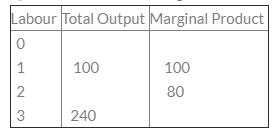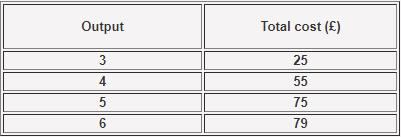Courses

# Test: Theory Of Production- 1

## 30 Questions MCQ Test Business Economics for CA Foundation | Test: Theory Of Production- 1

Description
This mock test of Test: Theory Of Production- 1 for CA Foundation helps you for every CA Foundation entrance exam. This contains 30 Multiple Choice Questions for CA Foundation Test: Theory Of Production- 1 (mcq) to study with solutions a complete question bank. The solved questions answers in this Test: Theory Of Production- 1 quiz give you a good mix of easy questions and tough questions. CA Foundation students definitely take this Test: Theory Of Production- 1 exercise for a better result in the exam. You can find other Test: Theory Of Production- 1 extra questions, long questions & short questions for CA Foundation on EduRev as well by searching above.
QUESTION: 1

### When output decreases by 20% due to increase in inputs by 20%, this stage called the law of _______

Solution:

By increasing inputs, output cannot decrease, so it is hypothetical statement and hence no law is present for this situation

QUESTION: 2

### Consider the following table:What is the total output, when 2 labour are employed?

Solution:

Marginal product is the result of total output of 2nd - total output of 1st. So let the total output of 2nd be x , then x-100=80, value of x is 180

QUESTION: 3

### Law of diminishing returns is applicable in _________

Solution:
QUESTION: 4

If a firm’s output is zero, then

Solution:

If the firm is operating at a level of output where the market price is at a level higher than the zero-profit point, then price will be greater than average cost and the firm is earning profits. If the price is exactly at the zero-profit point, then the firm is making zero profits.

QUESTION: 5

Production activity in the short run is analysed by

Solution:

The law of variable proportions which states marginal physical product of a variable factor eventually diminishes, even if it increases in the beginning.

QUESTION: 6Look carefully at the table which represents a firm's short-run total cost schedule. When output goes up from four to five shirts the marginal cost is:Solution:
QUESTION: 7

_________ shows the overall output generated at a given level of input :

Solution:
QUESTION: 8

At the point of inflexion, the marginal product is:

Solution:

At point of inflexion marginal product is maximum and here after it starts decreasing.

QUESTION: 9

Change in total revenue due to incremental change in quantity supplied is called:

Solution:
QUESTION: 10

If the marginal product of labour is below the average product of labour. It must be true that:

Solution:
QUESTION: 11

Increasing returns to scale can be explained in terms of:

Solution:
QUESTION: 12

Isoquants are equal to :

Solution:

An isoquant is a firm’s counterpart of the consumer’s indifference curve. An isoquant is a curve that shows all the combinations of inputs that yield the same level of output. ‘Iso’ means equal and ‘quant’ means quantity. Therefore, an isoquant represents a constant quantity of output. The isoquant curve is also known as an “Equal Product Curve” or “Production Indifference Curve” or Iso-Product Curve.”

QUESTION: 13

Given production is 1,00,000 units, fixed costs is Rs 2,00,000 Selling price is Rs 10 per unit and variable cost is Rs 6 per unit. Determine profit using technique of marginal costing.

Solution:
QUESTION: 14

Law of diminishing returns is applicable in :

Solution:
QUESTION: 15

What is Production in Economics:-

Solution:
QUESTION: 16

An Isoquant is ________ to an iso cost line at equilibrium point:

Solution:

Least Cost Factor Combination or Producer's Equilibrium or Optimal Combination of Inputs.The point of tangency between the isocost and an isoquant is an important but not a necessary condition for producer's equilibrium. The essential condition is that the slope of the isocost line must equal the slope of the isoquant .

QUESTION: 17

Innovation theory of entrepreneurship is propounded by

Solution:

Pronounced as one of the greatest economists of the 20th century, Joseph Alois Schumpeter breathed life into the concepts of innovation and entrepreneurship. According to his theory, innovation can be leveraged in: Launch of a new product or an upgraded version of an existing product.

QUESTION: 18

The concept of returns to scale is related with _________.

Solution:
QUESTION: 19

External economies can be achieved through:

Solution:

External economies of scale occur outside of a firm but within an industry.

For example investment in a better transport network servicing an industry will resulting in a decrease in costs for a company working within that industry

Investment in industry-related infrastructure including telecommunications can cut costs for all Another example is the development of research and development facilities in local universities that several businesses in an area can benefit from

Likewise, the relocation of component suppliers and other support businesses close to the centre of manufacturing are also an external cost saving.

Agglomeration economies may also result from the clustering of businesses in a distinct geographical location e.g. software in Silicon Valley or investment banks in the City of London

QUESTION: 20

Economies and diseconomies of scale explain why the:

Solution:

QUESTION: 21

The other names for capital formation is

Solution:

The other names for capital formation is investments

QUESTION: 22

Production activity in the short period is analysed with the help of

Solution:
QUESTION: 23

The marginal product curve is above the average product curve when the average product is:

Solution:
QUESTION: 24

Which one of the following is not a characteristic of land?

Solution:

Characteristics of land

Fixed Quantity:

Land is Permanent:

Land is a Primary Factor of Production

Land is a Passive Factor of Production

Land is Immovable

Land Differs in Fertility

Land has Many Uses
Hence, an active production is not a charterstic of land.

QUESTION: 25

Which of the following is not a characteristics of Land?

Solution:

Characteristics of land

Fixed Quantity:

Land is Permanent:

Land is a Primary Factor of Production

Land is a Passive Factor of Production

Land is Immovable

Land Differs in Fertility

Land has Many Uses

QUESTION: 26

With a view to increase his production, Hariharan a manufacturer of shoes, increases all the factors of production in his unit by 100%. But at the end of the year he finds that instead of an increase of 100%, his production has increased by only 80%. Which law of returns to scale is operating in this case?

Solution:
QUESTION: 27

External economies are enjoyed:

Solution:

The correct option is Option B.

Industry refers to a number of markets grouped together. So when an industry expands, there are a lot of sectors that are benefitted. All the firms in the economy get external economies i.e., they get the benefit of large scale business and they tend to grow further.

QUESTION: 28

Diminishing marginal returns implies :

Solution:

Diminishing returns to labour occurs when marginal product of labour starts to fall. This means that total output will be increasing at a decreasing rate. The law of diminishing returns implies that marginal cost will rise as output increases. Eventually, rising marginal cost will lead to a rise in average total cost.

QUESTION: 29

Law of variable proportion is valid when:

Solution:

The law of variable proportions come into being when there is a fixed factor and a variable factor. The law of variable proportions states that as the quantity of one factor is increased, keeping the other factors fixed, the marginal product of that factor will eventually decline.

QUESTION: 30

If LAC curve falls as output expands, this is due to ______:

Solution: